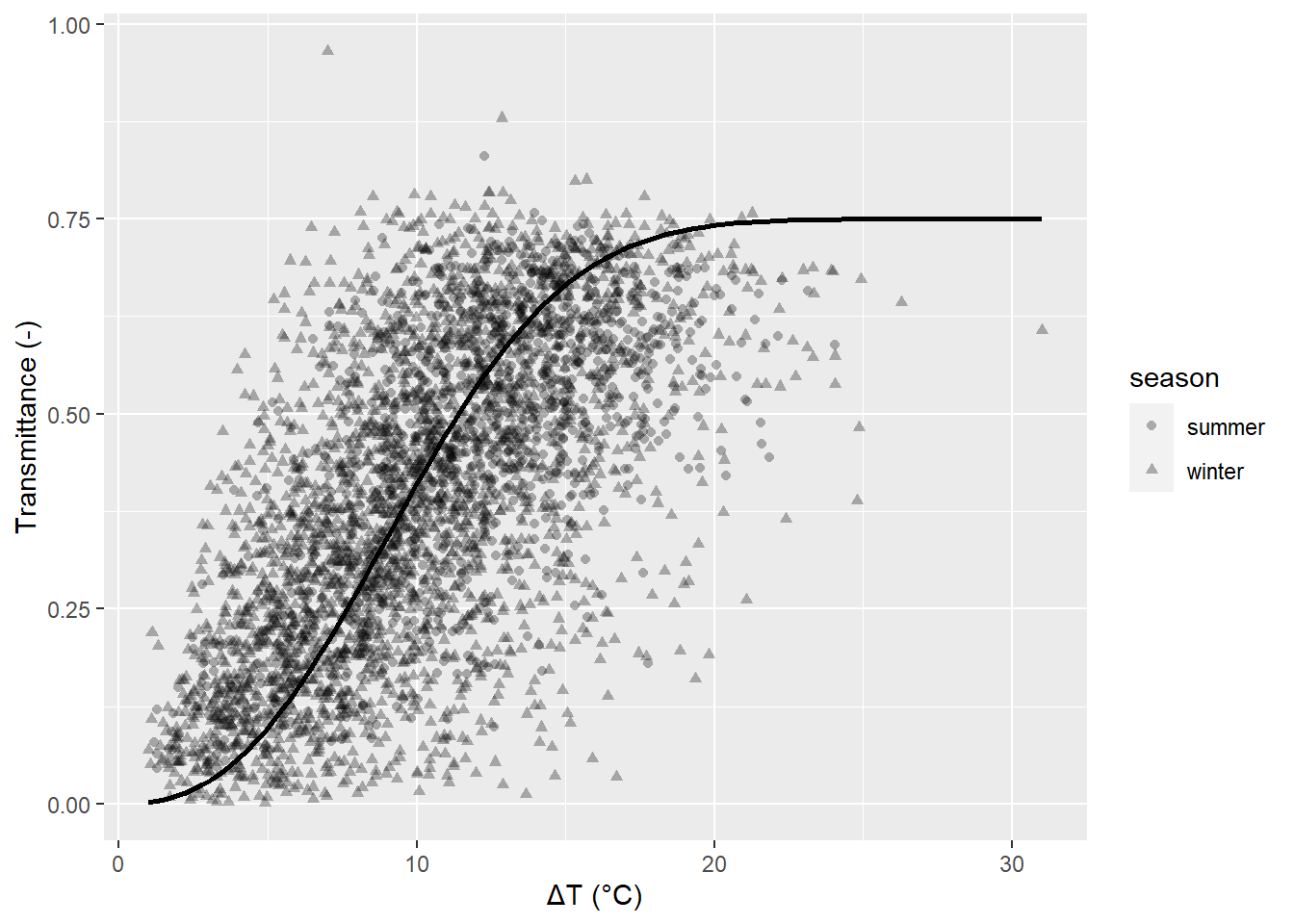## The Need

It is desirable to compute global radiation $$K_g$$ using extraterrestrial solar radiation $$K_e$$ approximated using solar irradiation theory and a combination readily available climate monitoring data such as temperatures and precipitation. While there are many methods to choose from (e.g., the Prescott-type equation; the Linacre (1992) equation; or the Hargreaves (1977; 1981) equations), here the Bristow and Campbell (1984) relationship is chosen as it is flexible and relies on temperature data:

$T_t = a\left[1 - e^{-b\Delta T^c}\right]$

where $$T_t$$ is transmittance, and $$a$$, $$b$$ and $$c$$ are empirical coefficients. $$K_g$$ is then approximated through the relationship:

$K_g=T_tK_e$

The coefficients $$a$$, $$b$$ and $$c$$ have difference somewhat physical interpretations: $$a$$ is the maximum clear sky transmittance, and can vary from 0.6 in smoggy air to 0.9 clean air and clear skies (Oke, 1987). $$b$$ and $$c$$ determine how quickly $$a$$ is achieved with increasing $$\Delta T$$; Bristow and Campbell (1984) kept $$c$$ constant as $$2.4$$ and adjusted $$b$$ to fit data.

As evident from below, there is a great deal of scatter in such data. Bristow and Campbell (1984) offered 3 “fixes” to the input data, none of which appeared to reduce the scatter, they included:

1. Set $$\Delta T(j)=T_\text{max}(j) - 0.5\left(T_\text{min}(j)+T_\text{min}(j+1)\right)$$ where $$j$$ is the day of observation. Here, Bristow and Campbell (1984) felt that relying on daily reported minimum and maximum temperatures caused some $$\Delta T$$ to be too excessive, and averaging the minimum temperatures before and after the daytime high helped reduce the noise. This did not appear to here, based on visual observation and thus $$\Delta T=T_\text{max} - T_\text{min}$$ was used;
2. On days with measured precipitation, set $$T_t=0.75T_t$$; and,
3. On days before a days with precipitation, where “$$\Delta T(j-1)$$ was less than $$\Delta T(j-2)$$ by more than 2°C”, set $$T_t(j-1)=0.75T_t(j-1)$$. This was to account for the assumption that cloud cover arrived a day before it rains on normally high-transmittance days.

## Validation

In replicating Figure 1 of Bristow and Campbell (1984), daily meteorological data from UTMMS between 1999 to 2010 was used, and a transmittance curve was visually fitted having the final parameter values of $$a=0.75$$, $$b=0.0025$$ and $$c=2.5$$: